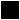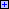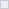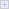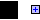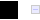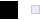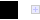# Neural Net Captcha Cracker

Geetika Garg, Chris Pollett (presenting)

San Jose State University

Future Technology Conference, San Francisco, Dec 6, 2016

# Purpose

Today, I'd like to report on work of myself and Geetika Garg on training neural networks to break image-based captchas.

# Agenda

• Prior Work
• Neural Nets
• Our Network
• Our Test Set
• Experiments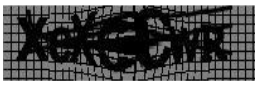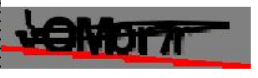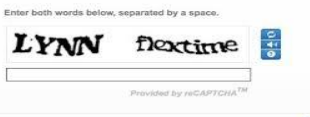• An image-based CAPTCHA, a Completely Automated Public Turing test to tell Computers and Humans Apart, is often used as part of a web form to prevent the form from being filled out by a web spam robot.
• It consists of an image with distorted text followed by a form field in which the text is required to be entered in order for the form to be processed.
• They are common enough that I am going to lower-case the acronym to captcha for the rest of the talk.
• Also, although there are non-image based captcha system, we will only consider image ones for this talk.
• Captcha-like systems were first proposed by Naor (1996), but were popularized by the paper of von Anh, et al (2003).
• They were first used commercially by Alta Vista and were patented in 2001.

# Prior Work

• There have been several reports on building automated tools for breaking captchas, for example, Mori and Malik (2003), Chellapilla and P. Y. Simard (2004).
• Another interesting related area is the recognition of street addresses and the development of street address datasets. (Netzer, Wang, et al 2011).
• These earlier system have some common steps which are different from the system we will discuss today:
1. Preprocessing
2. Segmentation
3. Training the model for individual character recognition
4. Generating sequence with highest probability
• Our system does not do the segmentation step, but operates on whole images instead.
• This results in a system that can better handle overlapping characters and the like.
• Our system also does not assume the captcha contains a fixed number of digits.

# What does it mean to break a Captcha?

• In real life, a human is allowed several tries to enter a captcha correctly.
• Suppose a breaker has a success rate of 50%.
• Allowed three tries, such a system's success chance jumps to $1 - (1-0.5)^3 = .875$.
• Many sites are based on the same open-source captcha systems.
• If the install base of the captcha system is a 100,000 sites, and the breaker tries once to post a spam message to each of these sites. It will succeed in posting 50,000 spam messages.
• Mori and Malik's breaker of the previous slide had a 92% success rate on EZGimpy captchas and a 33% rate on Gimpy captchas, so would be easily adequate to spread spam on sites using these kind of captchas.
• On the other hand, it wouldn't be sufficient if the target was a single large site and the goal was to post as many spam messages as possible before the large site banned the bot signature and ip.

# Artificial Neurons

• Our goal is to find a function which takes as input a $200 \times 50$, grayscale, captcha image and spits out the alphanumeric string contained in that input.
• We know humans are capable of computing this function.
• So rather than perform an undirected search through the space of possible functions from images to strings checking each function against our test set, we instead look for as small a subclass of functions as possible that enjoys enough similarities with the way humans solve captchas that it has a hope to contain the desired function.
• We then search this smaller space of functions for one that works on our test set.
• The human mind is built out of neurons, which as early as McCulloch and Pitts 1943, have been modeled mathematically in various ways.
• Our captcha breaker uses a couple of different models of artificial neurons, the easiest of which just computes a function $f(\vec{x}) = g(\sum_i w_i x_i)$. Here $x_i$ are the inputs to the neuron, $w_i$ are fixed, real-valued weights, and $g$ is an activation function, $g(t) = \max(0, t)$.
• When we train networks of neurons, we are trying to algorithmically adjust the weights $w_i$ for each neuron in the network so that the function computed by the network is as close to the desired function as possible.

# Neural Nets

• Neural networks are typically arranged into layers $L_1, \ldots, L_n$ of artificial neurons.
• In our case, the lowest layer receives inputs from the pixels of the captcha images.
• Thereafter, $L_{i+1}$, for the most part in a feedforward fashion, receives inputs only from layer $L_i$.
• The fewer the connections between the layers means the fewer the weights that needs to be determined in training, so training will go faster.
• People typically design their layers with this in mind, often using standard layer types, and the learning task at hand.

# Our Networks

• Two networks, a "multiple softmax network" and an "LSTM network", were built in Python using the Theano and Lasagne libraries, the latter extends the former and has an implementation of LSTM neurons that we also used.
• Both networks take input images and use a 5x5 filter CNN (Convolutional Neural Network) layer to produce 32 feature maps.
• Each 5x5 rectangle in the inputs corresponds serves as inputs to one neuron in a feature map. Neurons in a feature map all use the same weights. This is good for detecting simple features.
• These are downsampled using a 2x2 maxpool layer. (For each 2x2 rectangle in a feature map, maximum is output)
• A second CNN layer is applied to create the 32 downsampled maps and using a 5x5 filter to output 32 feature maps, which are again downsampled using a maxpool layer. (Try to detect higher order features.)
• In the "multiple softmax network", all the feature maps outputs are fed in parallel into five parallel, 256 neuron dense layers. The 256 outputs of these are fed into a softmax layer which, using a weighted sum of these, produces 62 final output signals, corresponding to the upper, lower, alpha or digit for that character in the captcha.
• In the "lstm network", rather than feed the dense layer into a softmax layer, the 256 dense layer outputs are fed into 256 long term short term (LSTM) units, a simple kind of recurrent network neuron, and the outputs of the LSTM layer are fed into the softmax layer as before.

# Inspiration for Our Networks

• Inspirations for our layer choices came from:
• LeCun, et al (1989) where CNN layers used in character recognition. They were in turn motivated by whole human visual system works.
• Goodfellow, el al (2014) CNN layers used for whole sequences of street addresses up to fixed length recaptchas (no segmentation).
• Vinyals, et al (2015) LSTM layers used to produce image captchas of various length.

# Our Test Set

• Training requires lots of images.
• A standard dataset for CAPTCHAs is not available publicly.
• We generated datasets synthetically using the BSD-licensed, open source, Simple CAPTCHA Java library.
• This library lets you generate CAPTCHAs with various kinds of noise, characters and backgrounds.
• We generated fixed as well as variable length CAPTCHA dataset.
• 1 million simple images (just straight line noise, clear background), 2 million complex images of fixed length 5 (straight or curved lined noise, variety of backgrounds and effects like fisheye-ing a portion of the image) , and 13 million complex images of variable length.
• Our code to generate our dataset is available at:
https://github.com/bgeetika/Captcha-Decoder/blob/master/training_data_gen/


# Training

• To learn the weights in our networks we used a variant of stochastic gradient descent (SGD), where the tweaks are well-known modifications to speed up convergence.
• As suggested by Haykin (2011), weights in our neurons were initially randomly chosen from a range $[-W_{bd}, W_{bd}]$ where $$W_{bd} = \big(\frac{12}{\mbox{num_neuron_in_out_edges}}\big)^{1/2}$$
• SGD typically computes the weights, $\vec{W}_{k+1,i}$, of $i$th layer after considering the $k$th training input output pair $(\vec{x}_k, \vec{y}_k)$ adjusts using a gradient of a loss function and backpropagation of errors from the final layer to earlier layers.
• We use the cross entropy function $$Loss(\vec{y}, \vec{x}, \vec{W}) := E(\vec{y}, \vec{f}(\vec{x}, \vec{W})) = \sum_m -y_m \ln {f_m(\vec{x}, \vec{W})}$$ where $\vec{f}$ will be the output of our net as our loss function.
• We clip gradients to the range $[-5, 5]$.
• To the basic SGD update we add a Nesterov Momentum term, so letting $\vec{\Delta}_{k+1, i} = \vec{W}_{k+1, i} - \vec{W}_{k,i}$, $\vec{W}_{k+1,i}$ is computed as $$\vec{W}_{k, i} - \lambda \frac{\partial \mbox{Loss}(\vec{y}, \vec{x},\vec{W})}{\partial\vec{W}_i}\Bigg{|}_{ \tiny{\begin{array}{c} \vec{y}= \vec{y}_k,\\ \vec{x}= \vec{x}_k,\\ \vec{W_i} =\vec{W}_{k,i}+ \mu\vec{\Delta}_{k,i} \end{array} } } + \mu \vec{\Delta}_{k, i}.$$
• To train our LSTM neurons which have edges which are not feedforward, we use a 5-fold forward unfolding version of backpropagation through time (BPTT).
• Training was done using Google Compute Engine with 8 cores and training times took from a couple days to up to a week depending on the training set.

# Experiments - Individual Characters

Type of modelIndividual Character Accuracy
LSTM fixed length (simple dataset)99.9%
LSTM fixed length (complex dataset)98.48%
Multiple Softmax fixed length (simple dataset)99.8%
Multiple Softmax fixed length (complex dataset)98.96%
LSTM variable length with fixed length data99.5%
LSTM variable length with variable length data97.31%

# Experiments - Sequence Correctness

Type of modelSequency Accuracy
LSTM fixed length (simple dataset)99.8%
LSTM fixed length (complex dataset)91%
Multiple Softmax fixed length (simple dataset)99%
Multiple Softmax fixed length (complex dataset)96%
LSTM variable length with fixed length data98%
LSTM variable length with variable length data81%

# Experiments - Versus Humans

• For fun we performed some experiments with three volunteers versus our neural net.
• Two volunteers tried to solve 10 randomly chosen complex captchas of variable length, a third volunteer did 15 captchas.
• On average the volunteers got about 26% of the captchas wrong.
• On the other hand on those same 30 captchas, our LSTM model got about 8% of the captchas wrong.

# Conclusion

• We have described our deep neural network decoding of image-based CAPTCHA experiments.
• Rather than the conventional approach of first cleaning a CAPTCHA image, segmenting the image, and recognizing the individual characters, our networks operate on the whole captcha image in one go.
• To train our models we developed a training set of 13 million images.
• Our sequence accuracy using LSTMs of 98% for fixed length captchas and 81% for variable length complex captchas, are better than earlier results such as Mori and Malik and Goodfellow, et al using comparably-hard CAPTCHA generators.

# References

(von Anh, et al 2003)
L. von Ahn, M. Blum. N. J. Hopper, and J. Langford. CAPTCHA: Using Hard AI Problems for Security. EUROCRYPT 2003: International Conference on the Theory and Applications of Cryptographic Techniques. 2003. pp. 294--311.
(Chellapilla, et al 2004)
K. Chellapilla and P. Y. Simard. Using Machine Learning to Break Visual Human Interaction Proofs (HIPs). Advances in Neural Information Processing Systems. Volume 17. NIPS 2004. pp. 265--272. 2004.
(Goodfellow, et al 2014)
I. J. Goodfellow, Y. Bulatov, J. Ibarz, S. Arnoud, V. Shet. Multi-digit Number Recognition from Street View Imagery using Deep Convolutional Neural Networks. International Conference on Learning Representations 2014. arXiv:1312.6082. 2014.
(Haykin 2011)
S. O. Haykin. Neural Networks and Learning Machines. 3rd Ed. Pearson. 2011.
(LeCun, et al 1989)
Y. LeCun, B. Boser, J. S. Denke, D. Henderson, R. E. Howard, W. Hubbard and L. D. Jackel. Handwritten digit recognition with a back-propagation network. Advances in Neural Information Processing Systems. Volume 2. NIPS 1989. pp. 396--404. 1989.
(McCulloch and Pitts 1943)
S. McCulloch and W. H. Pitts. Resource Description for A logical calculus of the ideas immanent in nervous activity. Bulletin of Mathematical Biophysics. Volume 5. pp. 115--133. 1943.
(Mori and Malik 2003)
G. Mori and J. Malik. Recognizing Objects in Adversarial Clutter: Breaking a Visual CAPTCHA. IEEE Conference on Computer Vision and Pattern Recognition. CVPR 2003. Vol. 1. IEEE Computer Society. pp.134--141. 2003.
(Naor 1996)
M. Naor. Verification of a Human in the Loop, or Identification via the Turing Test. Unpublished Manuscript. 1996.
(Netzer, et al 2011)
Yuval Netzer, Tao Wang, Adam Coates, Alessandro Bissacco, Bo Wu, Andrew Y. Ng Reading Digits in Natural Images with Unsupervised Feature Learning NIPS Workshop on Deep Learning and Unsupervised Feature Learning 2011.
(Vinyals, et al 2015)
O. Vinyals, A. Toshev, S. Bengio, and D. Erhan. Show and Tell: A Neural Image Caption Generator. IEEE Conference on Computer Vision and Pattern Recognition. pp. 3156--3164. 2015.